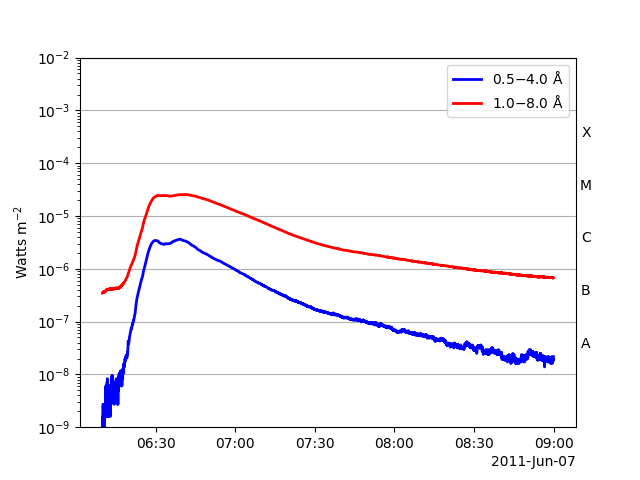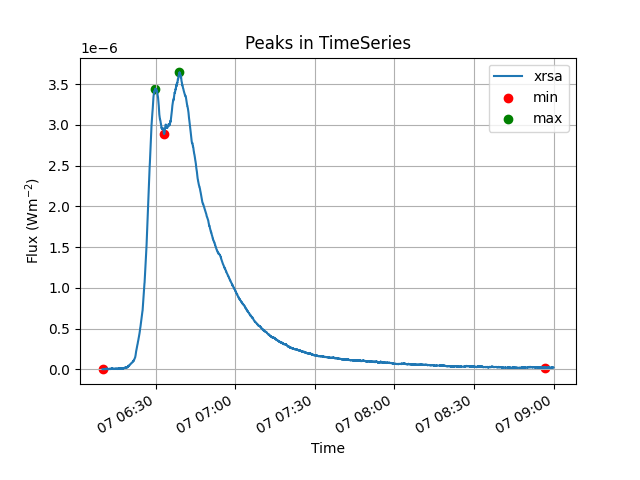# Find Peaks in sunpy TimeSeries#

This example illustrates how to find minimum or maximum peaks in a TimeSeries. Note: Peak finding is a complex problem that has many potential solutions and this example is just one method of many.

import matplotlib.pyplot as plt
import numpy as np

from sunpy.data.sample import GOES_XRS_TIMESERIES
from sunpy.timeseries import TimeSeries


We will now create a TimeSeries object from an observational data source, Also, we will truncate it to do analysis on a smaller time duration of 10 years.

goes_lc = TimeSeries(GOES_XRS_TIMESERIES)
my_timeseries = goes_lc.truncate('2011/06/07 06:10', '2011/06/07 09:00')

fig, ax = plt.subplots()
my_timeseries.plot(axes=ax)<Axes: ylabel='Watts m$^{-2}$'>


To find extrema in any TimeSeries, we first define a function findpeaks that takes in input an iterable data series and a DELTA value. The DELTA value controls how much difference between values in the TimeSeries defines an extremum point. Inside the function, we iterate over the data values of TimeSeries and consider a point to be a local maxima if it has the maximal value, and was preceded (to the left) by a value lower by DELTA. Similar logic applies to find a local minima.

def findpeaks(series, DELTA):
"""
Finds extrema in a pandas series data.

Parameters
----------
series : pandas.Series
The data series from which we need to find extrema.

DELTA : float
The minimum difference between data values that defines a peak.

Returns
-------
minpeaks, maxpeaks : list
Lists consisting of pos, val pairs for both local minima points and
local maxima points.
"""
# Set initial values
mn, mx = np.Inf, -np.Inf
minpeaks = []
maxpeaks = []
lookformax = True
start = True
# Iterate over items in series
for time_pos, value in series.items():
if value > mx:
mx = value
mxpos = time_pos
if value < mn:
mn = value
mnpos = time_pos
if lookformax:
if value < mx-DELTA:
# a local maxima
maxpeaks.append((mxpos, mx))
mn = value
mnpos = time_pos
lookformax = False
elif start:
# a local minima at beginning
minpeaks.append((mnpos, mn))
mx = value
mxpos = time_pos
start = False
else:
if value > mn+DELTA:
# a local minima
minpeaks.append((mnpos, mn))
mx = value
mxpos = time_pos
lookformax = True
# check for extrema at end
if value > mn+DELTA:
maxpeaks.append((mxpos, mx))
elif value < mx-DELTA:
minpeaks.append((mnpos, mn))
return minpeaks, maxpeaks


Now we take the column ‘sunspot SWO’ of this TimeSeries and try to find it’s extrema using the function findpeaks. We take the value of DELTA to be approximately the length of smallest peak that we wish to detect.

series = my_timeseries.to_dataframe()['xrsa']
minpeaks, maxpeaks = findpeaks(series, DELTA=1e-7)

# Plotting the figure and extremum points
fig, ax = plt.subplots()
ax.set_xlabel('Time')
ax.set_ylabel("Flux (Wm$^{-2}$)")
ax.set_title('Peaks in TimeSeries')
series.plot(ax=ax)
ax.scatter(*zip(*minpeaks), color='red', label='min')
ax.scatter(*zip(*maxpeaks), color='green', label='max')
ax.legend()
ax.grid(True)

plt.show()Total running time of the script: (0 minutes 0.703 seconds)

Gallery generated by Sphinx-Gallery